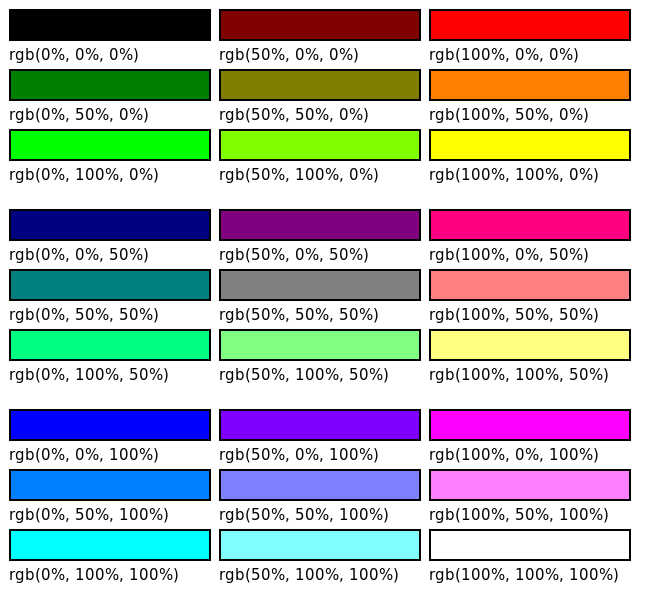# RGB colour space

By Martin McBride, 2021-04-25
Tags: colour rgb
Categories: computer science colourAs we saw in light and colour, colour can be represented by 3 values, red, green and blue. Each unique combination of red, green and blue creates a unique colour.

You could think of these values as axes in a 3 dimensional space, where each point in space is a particular colour. We call this space the RGB colour space.

We say that an RGB colour has 3 components - red, green and blue.

## Displaying colour

In a computer screen, each pixel has three tiny elements (typically LCD cells that can be made transparent or opaque by an electrical signal). These act as a variable source of red, green and blue light. By illuminating these sources by the correct amount, we can set each pixel to any colour.

We can store the required colour of each pixel as 3 numbers in the computers video memory. This data control the intensity of each colour.

## Representing RGB colours as a percentage

There are several ways to represent an RGB colour, but they all amount to the same thing: a colour is specified by three numbers, that represent the amounts of red, green and blue that make up that colour.

The first way is use a percentage, so for the red value:

• 0% means that the colour has no red component. For example pure blue has no red component, and pure black has no colour at all.
• 100% means that the maximum possible amount of that component is present. For example the brightest pure red contains the maximum possible amount of red, and the brightest white contains the maximum possible amount of all three components
• 50% means that the colour contains half the maximum amount of red.

Similar for green and blue.

We can specify any RGB colour using percentages, like this:

rgb(100%, 50%, 0%)


This indicates a colour that contains the maximum amount of red. 50% of the maximum green, and no blue. That would give an orange colour.

Here are a selection of example colours:This table shows every combination of red, green and blue values of 0%, 50% and 100%.

## Floating point representation

An alternative way to represent an RGB colour is to use numbers in the range 0.0 to 1.0. This works in exactly the same way as percentages, but with fractions instead of percentages:

• A value of 0.0 corresponds to 0%.
• A value of 1.0 corresponds to 100%.
• A value of 0.5 corresponds to 50% and so on.

So the value:

rgb(100%, 50%, 0%)


would be represented by three floating point values (1.0, 0.5, 0.0).

Pycairo represents colours in this way, and NumPy sometimes uses this method to store images.

## Byte value representation

The final method is to represent each colour channel as an integer value between 0 and 255:

• A value of 0 corresponds to 0%.
• A value of 128 corresponds to 100%.
• A value of 255 corresponds to 50% and so on.

So the value:

rgb(100%, 50%, 0%)


would be represented by three integer values (255, 128, 0).

The reason we choose the range 0 to 255 is because that is the range that can be stored in an unsigned byte. This means that a colour can be stored in exactly 3 bytes of memory, and an image can be stored using 3 bytes per pixel.

It turns out that 256 levels of each colour is enough to be able to represent colours precisely enough for most purposes - it is good enough for photographs and videos, for example. This is covered more in colour depth.